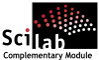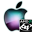ATOMS : Apifun details

# Apifun

Check input arguments in macros.Details
Version
0.1
A more recent valid version with binaries for Scilab 5.2 exists: 0.3
Author
Michael Baudin
Owner Organization
DIGITEO
Maintainers
Michael BAUDIN
Allan CORNET
Category
Creation Date
August 6, 2010
Source created on
Scilab 5.2.x
Binaries available on
Scilab 5.2.x:Windows 64-bitWindows 32-bitLinux 64-bitLinux 32-bitmacOS
Install command
`--> atomsInstall("apifun")`
Report a bugDescription
```            Purpose

The goal of this module is to provide a set to function to check input arguments
in macros.

In functions with a variable number of input arguments,
we often have the following source code, which checks that
the number of input arguments provided by the user is
consistent with the number of expected arguments :

[lhs,rhs]=argn()
if ( rhs < 2 | rhs > 5 ) then
errmsg = msprintf(gettext("%s: Unexpected number of input arguments : %d
provided while from %d to %d are expected."), "myfunnction", rhs,2,5);
error(errmsg)
end

Writing this code is error-prone and boring.
Moreover, this fragment of source code is duplicated in
many functions, leading to small variations depending on the
developer.
In particular, this makes the localization of the error messages
difficult, because the message can be slightly different
depending on the function.
It can also lead to unexpected errors, for example when the
string containing the error message is wrongly formatted.
In this case, instead of the expected error, the user gets a message telling
that the argument of the msprintf function has a wrong format ; this
is an information which can let the user confused about the source
of the error.
Moreover, because writing this source code is boring, many existing functions
are not sufficiently robust against wrong uses.

The goal of the apifun module is to simplify this task.
For example, the apifun_checkrhs function checks that the number of
input arguments provided by the user of the function corresponds
to the number of expected arguments.
The following function takes 2/3 input arguments and 1 output arguments.

function y = myfunction ( varargin )
[lhs, rhs] = argn()
apifun_checkrhs ( "myfunction" , rhs , 2:3 )
apifun_checklhs ( "myfunction" , lhs , 1 )
x1 = varargin(1)
x2 = varargin(2)
if ( rhs >= 3 ) then
x3 = varargin(3)
else
x3 = 2
end
y = x1 + x2 + x3
endfunction

When called with 1 or 4 arguments, an error will be
generated.

// Calling sequences which work
y = myfunction ( 1 , 2 )
y = myfunction ( 1 , 2 , 3 )
// Calling sequences which generate an error
y = myfunction ( 1 )
y = myfunction ( 1 , 2 , 3 , 4 )

The following session shows the kind of error message which is
produced by the apifun module, for example when the number of input argument is
wrong.
The error message is clear about the function which generates the message (e.g.
"myfunction").

-->y = myfunction ( 1 )
!--error 10000
myfunction: Unexpected number of input arguments : 1 provided while the number
of expected input arguments should be in the set [2 3].
at line     131 of function apifun_checkrhs called by :
at line       3 of function myfunction called by :
y = myfunction ( 1 )

The advantages of using the current module are the following.
* Designing robust functions is much simpler which improves both the
quality and the robustness of functions.
* The provided source code is factored into one single
module.
* It is similar to what is provided to the developers of Scilab gateways,
at the C level.

The following is a list of the function currently implemented in this module:
apifun_checkdims : Generates an error if the variable has not the required
size.
apifun_checkgreq : Check that the value is greater or equal than a threshold.
apifun_checklhs : Generates an error if the number of LHS is not in given set.
apifun_checkloweq : Checks that the value is lower or equal than a threshold.
apifun_checkoption : Generates an error if the value of an input argument is not
expected.
apifun_checkrange : Check that the value is in a given range.
apifun_checkrhs : Generates an error if the number of RHS is not in given set.
apifun_checkscalar : Generates an error if the variable is not a scalar.
apifun_checksquare : Generates an error if the variable is not a square matrix.
apifun_checktype : Generates an error if the given variable is not of expected
type.
apifun_checkveccol : Generates an error if the variable is not a column vector.
apifun_checkvecrow : Generates an error if the variable is not a row vector.
apifun_checkvector : Generates an error if the variable is not a vector.

```Files (2)Source code archive
```
```OS-independent binary for Scilab 5.2.x
```Binary version
Automatically generated by the ATOMS compilation chain

```News (0)Comments (0)Leave a comment
Login withEmail notifications